# How to Use qnorm() function in R

The qnorm() function is used to calculate quantiles of the standard normal distribution (also known as the Z-distribution). It accepts a probability (or probabilities) as input and returns the corresponding quantiles from the standard normal distribution.

## Syntax

``````qnorm(p, mean = 0, sd = 0, lower.tail = TRUE)
``````

## Parameters

1. p: It represents the significance level to be used
2. mean: It is a vector of means.
3. sd: It is a vector of standard deviation.
4. lower.tail = TRUE: It is a probability to the left of p in the normal distribution that is returned.

## Example 1: How to Use qnorm() function

Let’s say you want to find the Z-value for a probability of 0.90 (90% confidence level):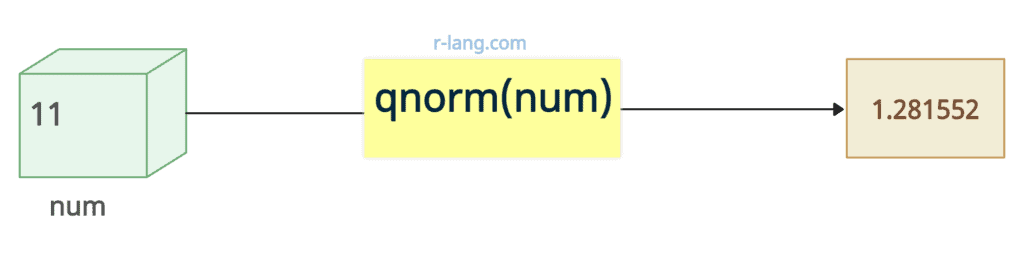``````# Calculate the Z-value for a 90% confidence level
z_value <- qnorm(0.90)

z_value``````

Output

`````` 1.281552
``````

Let’s generate a complete plot of the standard normal distribution with a vertical red line indicating the Z-value for a 90% confidence level and a label for that Z-value.

``````# Calculate the Z-value for a 90% confidence level
z_value <- qnorm(0.90)

# Create a sequence of x values
x <- seq(-3, 3, by = 0.01)

# Calculate the probability density function (PDF)
# values for the standard normal distribution
pdf_values <- dnorm(x)

# Create a plot
plot(x, pdf_values,
type = "l",
main = "Standard Normal Distribution",
xlab = "Z-value", ylab = "PDF"
)

# Add a vertical line at the Z-value for the 90% confidence level
abline(v = z_value, col = "red", lwd = 2)

# Add a text label to the vertical line
text(z_value + 0.1, max(pdf_values) * 0.8,
labels = round(z_value, 2),
col = "red"
)``````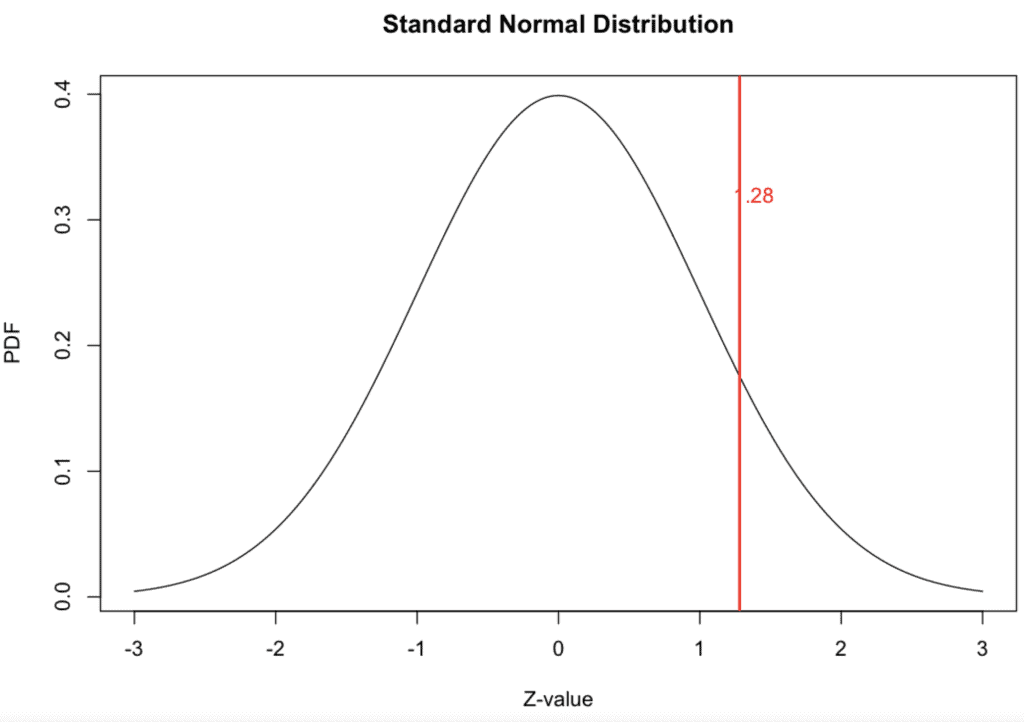You can see the complete plot of the standard normal distribution with a vertical red line suggesting the Z-value (1.28) for a 90% confidence level and a label for that Z-value (1.28). The value in the graph is 1.28, but the vertical line overlaps it.

## Example 2: Finding Multiple Z-values for Given Probabilities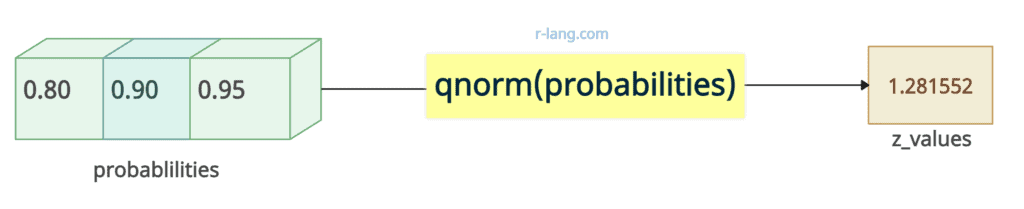``````# Calculate Z-values for 80%, 90%, and 95% confidence levels
probabilities <- c(0.80, 0.90, 0.95)

z_values <- qnorm(probabilities)

z_values``````

Output

`````` 1.281552
``````

Let’s plot the graph.

``````# Calculate Z-values for 80%, 90%, and 95% confidence levels
probabilities <- c(0.80, 0.90, 0.95)
z_values <- qnorm(probabilities)

# Create a bar plot to display the Z-values
barplot(z_values, names.arg = c("80%", "90%", "95%"),
main = "Z-values for Confidence Levels",
ylab = "Z-values", col = "light blue")

# Add text labels to the bars with adjusted 'pos' for the 95% label
text(1:3, z_values, labels = round(z_values, 2), pos = c(3, 3, 1), col = "red")``````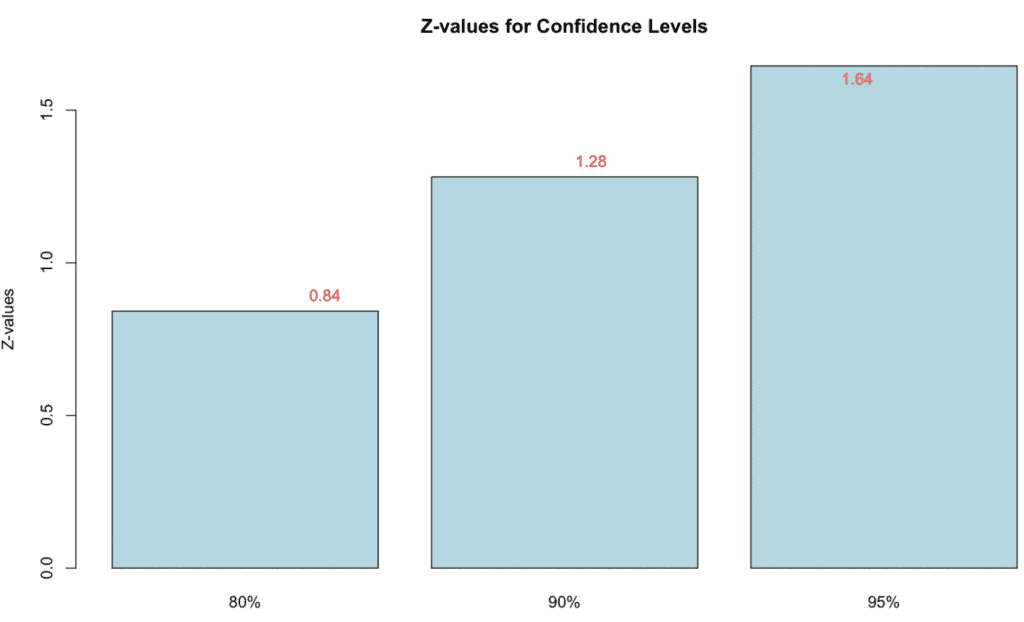We created a barplot showing the Z-values for the 80%, 90%, and 95% confidence levels obtained from the qnorm() function.

The text() function labels the bars with the corresponding Z-values, rounded to two decimal places.

## Example 3: Calculating Z-values for Quantiles of a Normal Distribution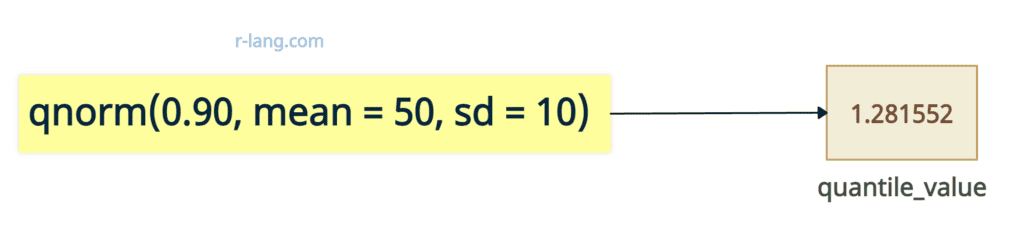Let’s find the 90th percentile of a normal distribution with a mean of 50 and a standard deviation of 10.

``````# Calculate the Z-value for the 90th percentile of N(50, 10^2)
mean_value <- 50

sd_value <- 10

quantile_value <- qnorm(0.90, mean = mean_value, sd = sd_value)

quantile_value``````

Output

`````` 1.281552
``````

Let’s plot a graph based on the above output.

``````# Calculate the Z-value for the 90th percentile of N(50, 10^2)
mean_value <- 50
sd_value <- 10
quantile_value <- qnorm(0.90, mean = mean_value, sd = sd_value)

# Create a bar plot to display the quantile value
barplot(quantile_value,
names.arg = "90th Percentile",
main = "Z-value for 90th Percentile",
ylab = "Z-value", col = "blue"
)

# Add text label to the bar
text(1, quantile_value,
labels = round(quantile_value, 2), pos = 2, col = "black"
)
``````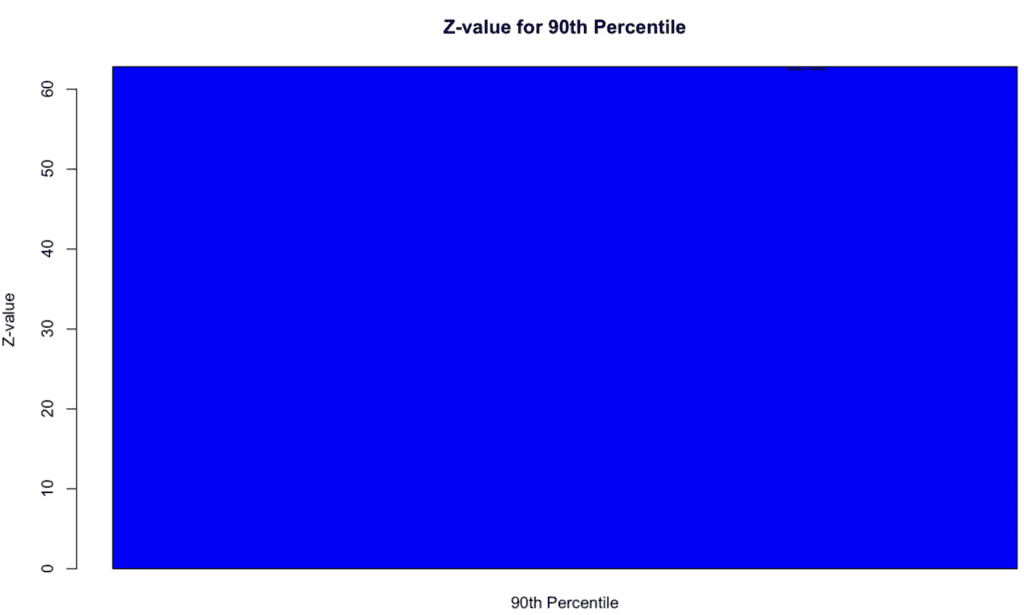You can see a barplot with a single bar representing the Z-value for the 90th percentile of the specified normal distribution.

The text() function labels the bar with the Z-value, rounded to two decimal places.

rnorm() function

pnorm() function

dnorm() function